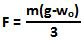## Thursday, December 11, 2008

### Irodov Problem 1.254This problem is identical in all respects to problem 1.253 except for the fact this is inside an accelerating elevator. Using the famous Einstien's principle of equivalence, with respect to an observer in the accelerating elevator, it will be as if the net gravitational attraction inside the elevator has become g-wo. Thus, the solution for this problem is exactly the same as that of 1.253 except that the acceleration due to gravity g will be replaced by g-wo.

Thus, based on eqn (5) in 1.253, in this problem the acceleration as seen by the observer in the elevator will be,The force F exerted on the elevator the combined tensions in the two strings 2T. So replacing g by g-wo in eqn (6) we have,